# Lebesgue-Stieltjes integral

(diff) ← Older revision | Latest revision (diff) | Newer revision → (diff)

A generalization of the Lebesgue integral. For a non-negative measurethe name "Lebesgue–Stieltjes integral" is used in the case when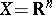andis not the Lebesgue measure; then the integral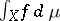is defined in the same way as the Lebesgue integral in the general case. Ifis of variable sign, then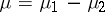, where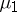andare non-negative measures, and the Lebesgue–Stieltjes integral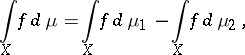under the condition that both integrals on the right-hand side exist. For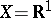the fact thatis countably additive and bounded is equivalent to the fact that the measure is generated by some functionof bounded variation. In this case the Lebesgue–Stieltjes integral is written in the formFor a discrete measure the Lebesgue–Stieltjes integral is a series of numbers.

How to Cite This Entry:
Lebesgue-Stieltjes integral. Encyclopedia of Mathematics. URL: http://encyclopediaofmath.org/index.php?title=Lebesgue-Stieltjes_integral&oldid=14842
This article was adapted from an original article by I.A. Vinogradova (originator), which appeared in Encyclopedia of Mathematics - ISBN 1402006098. See original article